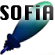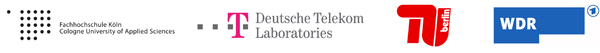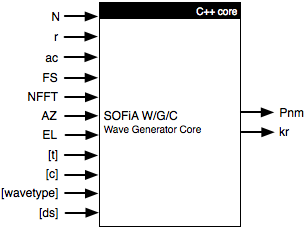Welcome to SOFiA
Who is behind SOFiA
Feature overview
System overview
Function reference
mergeArrayData
F/D/T
gauss
lebedev
S/W/G
S/T/C
W/G/C
S/F/E
M/F
R/F/I
P/D/C
I/T/C
makeMTX
makeIR
visual3D
Coordinate System
Application Examples
Example 1
Example 2
Example 3
Example 4
Example 5
Example 6
Example 7
Example 8
Array Datasets
VariSphear system
Groups and Mailinglists
Contact and Support
How to Reference

# SOFiA Wave Generator Core

The W/G/C Wave Generator Core delivers ideal analytical fourier coefficients emulating a full spectrum (0-FS/2) wave impact to an array.## ARGUMENTS

Input

Name Type Purpose Default
N int Maximum order -
r float Microphone radius in m
Can also be a vector for rigid/dual sphere configurations:
! If only one radius (rm) is given using a Rigid/Dual Sphere Configuration:
rs = rm. In this case only one kr-vector is returned.
-
ac int

Array configuration
0: Open Sphere with p Transducers
1: Open Sphere with pGrad Transducers
2: Rigid Sphere with p Transducers
3: Rigid Sphere with pGrad Transducers
4: Dual Open Sphere with p Transducers

-
FS int

Sampling rate

-
NFFT int

FFT Order (Number of bins) should be 2^x, x=1,2,3,…

-
AZ float

Azimuthal angle of the wave (Refer to Coordinate System)

-
EL float

Elevation angle of the wave (Refer to Coordinate System)

-
t float

Time delay in s

0
c float

Speed of sound in m/s

343.0
wavetype int

0: Plane wave, 1: Spherical wave

0
ds float

Source distance in m (For wavetype = 1, spherical wave only)
WARNING: If NFFT is smaller than the timer the wavefront needs
to travel from the source to the array, the impulse response will by
cyclically shifted (cyclic convolution)

1m

Angles AZ, EL are in RAD

Output

Name Type Purpose
fftData complexfloat mtx Frequency domain data for S/T/C
kr float vec [1xNFFT/2+1]or
float mtx[2xNFFT/2+1]
kr-Vector (k: wave number, r: radius)
Can also be a matrix krs for rigid sphere configurations:
(1,:) =>krm referring to the Microphone Radius
(2;:) =>krs referring to the Sphere/Microphone2 Radius

## FILE(S):

 File Type OS/Matlab sofia_wgc.m Help header All OS sofia_wgc.mexmaci64 MEX Core OS-X Intel sofia_wgc.mexw32 MEX Core Windows, MATLAB32 sofia_wgc.mexw64 MEX Core Windows, MATLAB64 sofia_wgc.cpp C/C++ source All OS sofia_radial.h internal C/C++ header All OS sofia_radial.cpp internal C/C++ header/source All OS

 ```/* [Pnm, kr] = sofia_wgc(N, r, ac, FS, NFFT, AZ, EL,...                 t, c, wavetype, ds, lSegLim, uSegLim, SeqN)``` `------------------------------------------------------------------------------------` `Pnm` ```Spatial Fourier Coefficients Columns: nm coeff Rows: FFT bins``` ``` kr``` ``` kr-Vector Can also be a matrix [krm; krs] for rigid sphere configurations: [1;:] => krm referring to the Microphone Radius [2;:] => krs referring to the Sphere/Microphone2 Radius``` `------------------------------------------------------------------------------------` `N` `Maximum transform order` `r` ```Microphone Radius Can also be a vector for rigid sphere configurations: [1,1] => rm Microphone Radius [2,1] => rs Sphere Radius (Scatterer) ``` ``` q_grid``` ``` Quadrature grid [default LEB110] Columns: Position Number 1...M Rows: [AZ EL Weight] Angles AZ, EL in [RAD] ``` ``` ac``` ``` Array Configuration 0 Open Sphere with p Transducers 1 Open Sphere with pGrad Transducers 2 Rigid Sphere with p Transducers 3 Rigid Sphere with pGrad Transducers (Thx to Nils Peters!) 4 Dual Open Sphere with p Transducers (Thx to Nils Peters!)``` `FS` `Sampling Frequency` `NFFT` `FFT Order (Number of bins) shhould be 2^x, x=1,2,3,...` `AZ` `Azimuth angle in [DEG] 0-2pi` `EL` `Elevation angle in [DEG] 0-pi` `c` `Speed of sound in [m/s] (Default: 343m/s)` `t` `Time Delay in s` `wavetype` `Type pf the Wave. 0: Plane wave (default); 1: Spherical Wave` `ds` ```Distance of the source in [m] (For wavetype = 1 only) Warning: If NFFT is smaller than the time the wavefront needs to travel from the source to the array, the impulse response will by cyclically shifted (cyclic convolution).``` `lSegLim` `(Lower Segment Limit) Used by the S/W/G wrapper` `uSegLim` `(Upper Segment Limit) Used by the S/W/G wrapper` `SegN` `(Segment Order)       Used by the S/W/G wrapper` `*/`